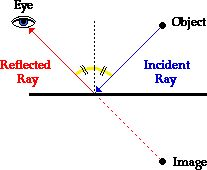# Two plane mirrors are inclined to each other such that a ray of light incident on the first mirror (M1) and parallel to the second mirror (M2) is finally reflect from the second mirror (M2) parallel to the first mirror (M1). The angle between the two mirrors will be:Option 1)Option 2)Option 3)Option 4)

Law of reflection -

1)    Incident ray, reflected rays and normal at the point of incidence lie in the same plane.

2)    Angle of incidence is equal to angle of reflection.

- whereinOption 1)

Option 2)

Option 3)

Option 4)

Exams
Articles
Questions